MATLAB ProblemsPlease read this before your start *Use MATLAB to solve these problems. *Submit your answers any time before 7:00AM Sunday June 7, 2009 *Here is a  quick start to MATLAB *Let    w = ( last five digits of your ID number ) / 10000  (Example: ID=200612340  then  w=12340/10000=1.234) *Be aware that each student has different answers. *To submit    3.567 X 10^(-33)    type     3.567e-33    (round to 3 decimal digits) *First solve all the 8 problems then enter all the 8 answers in the left page then click on submit button. * Here is an example ( How to enter the numbers ) *After you click on Submit button, wait until you receive the confirmation page. *practice before you submit ( ppt, pdf )

1)

Let E be the reduced-row echelon form of the matrix A whereThen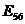=  ??

2)

Let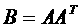then   det( B ) = ????

3)

Letand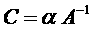then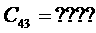4)

Let :   (how many negative eigenvalues)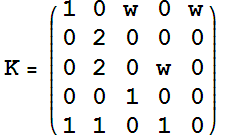Then the matrix  K  has   -------   negative eigenvalues.

5)

Find the characteristic equation of the matrix  K   then compute:6)

Let: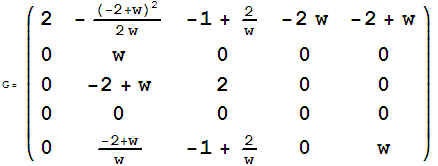Determine whether  or not the matrix G is diagonalizable

7)

Solve the IVP:Then find the value the solution at x=1.   y(1)=????

8)

Solve the following linear system of differential equations:Then find:    x(2) = ?????